Next: Motion in a Central Up: Lagrangian Dynamics Previous: Generalized Forces

Lagrange's Equation

The Cartesian equations of motion of our system take the form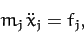(600)

for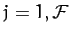, where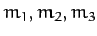are each equal to the mass of the first particle,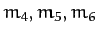are each equal to the mass of the second particle, etc. Furthermore, the kinetic energy of the system can be written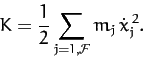(601)

Now, since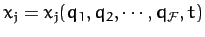, we can write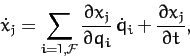(602)

for. Hence, it follows that. According to the above equation,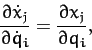(603)

where we are treating the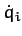and the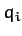as independent variables.

Multiplying Equation (603) by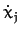, and then differentiating with respect to time, we obtain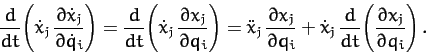(604)

Now,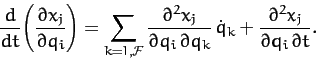(605)

Furthermore,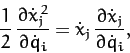(606)

and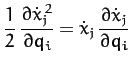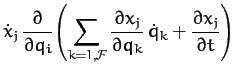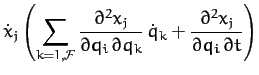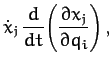(607)

where use has been made of Equation (605). Thus, it follows from Equations (604), (606), and (607) that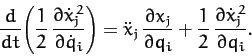(608)

Let us take the above equation, multiply by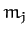, and then sum over all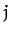. We obtain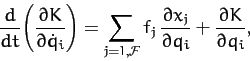(609)

where use has been made of Equations (600) and (601). Thus, it follows from Equation (597) that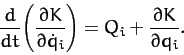(610)

Finally, making use of Equation (599), we get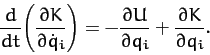(611)

It is helpful to introduce a function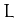, called the Lagrangian, which is defined as the difference between the kinetic and potential energies of the dynamical system under investigation: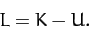(612)

Since the potential energy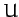is clearly independent of the, it follows from Equation (611) that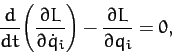(613)

for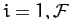. This equation is known as Lagrange's equation.

According to the above analysis, if we can express the kinetic and potential energies of our dynamical system solely in terms of our generalized coordinates and their time derivatives then we can immediately write down the equations of motion of the system, expressed in terms of the generalized coordinates, using Lagrange's equation, (613). Unfortunately, this scheme only works for conservative systems. Let us now consider some examples.Next: Motion in a Central Up: Lagrangian Dynamics Previous: Generalized Forces
Richard Fitzpatrick 2011-03-31